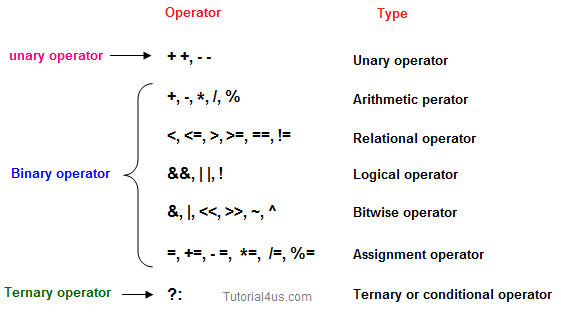# Operators

## Operators

Operator is a special symbol that tells the compiler to perform specific mathematical or logical Operation.

• Arithmetic Operators
• Relational Operators
• Logical Operators
• Bitwise Operators
• Assignment Operators
• Ternary or Conditional Operators## Arithmetic Operators

Given table shows all the Arithmetic operator supported by C Language. Lets suppose variable A hold 8 and B hold 3.

OperatorExample (int A=8, B=3)Result
+A+B11
-A-B5
*A*B24
/A/B2
%A%40

## Relational Operators

Which can be used to check the Condition, it always return true or false. Lets suppose variable A hold 8 and B hold 3.

OperatorsExample (int A=8, B=3)Result
<A<BFalse
<=A<=10True
>A>BTrue
>=A<=BFalse
==A== BFalse
!=A!=(-4)True

## Logical Operator

Which can be used to combine more than one Condition?. Suppose you want to combined two conditions A<B and B>C, then you need to use Logical Operator like (A<B) && (B>C). Here && is Logical Operator.

OperatorExample (int A=8, B=3, C=-10)Result
&&(A<B) && (B>C)False
||(B!=-C) || (A==B)True
!!(B<=-A)True

## Truth table of Logical Operator

C1C2C1 && C2 C1 || C2!C1!C2
TTTTFF
TFFTFT
FTFTTF
FFFFTT

## Assignment operators

Which can be used to assign a value to a variable. Lets suppose variable A hold 8 and B hold 3.

OperatorExample (int A=8, B=3)Result
+=A+=B or A=A+B11
-=A-=3 or A=A+35
*=A*=7 or A=A*756
/=A/=B or A=A/B2
%=A%=5 or A=A%53
=a=bValue of b will be assigned to a